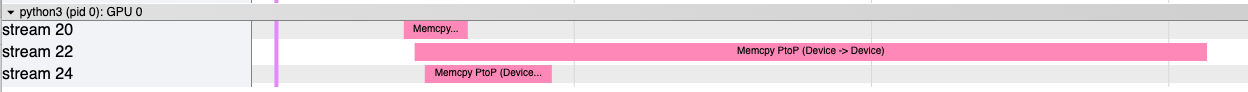## Puzzler 1: Peer to Peer Bandwidth

Server topology

The figure above shows the network topology of the GPUs in a server. As observed from the trace, the data_transfer function copies a list of tensors from GPU 0 to GPU 1, GPU 2 and GPU 4 in 4.3 ms, 53.3 ms and 8.5 ms respectively. Why do the peer to peer copies to different GPUs vary so much?Trace for data_transfer function

def data_transfer():
with torch.cuda.stream(first):
data.to(torch.device('cuda:1'))

with torch.cuda.stream(second):
data.to(torch.device('cuda:2'))

with torch.cuda.stream(third):
data.to(torch.device('cuda:4'))

first, second, third = [torch.cuda.Stream() for _ in range(3)]
cuda = torch.device('cuda:0')
data = torch.rand((10**8), device = cuda, dtype = torch.float32)


## Puzzler 2: Collective Performance

Message Passing Interface (MPI) is a communication protocol used in parallel computing. It supports both point-to-point (Send and Receive) and collective (Reduce, Broadcast, Scatter, Gather, All_Reduce etc.) communication. PyTorch DDP and FSDP use MPI collectives under the hood to do distributed training.

It is well known that All_Reduce is mathematically equivalent to Reduce followed by Broadcast. With some thought one can prove that All_Reduce is equivalent to Reduce_Scatter followed by All_Gather.

The functions below use All_Reduce, Reduce + Broadcast, and Reduce_Scatter + All_Gather to sum tensors. What are the factors influencing the performance of the three implementations?

# Approach 1 - call All_Reduce directly.
def all_reduce_sum(size, local_rank, tensor_size=2**20):
group = torch.distributed.new_group(list(range(size)))
device = torch.device(f"cuda:{local_rank}")
tensor = torch.rand(tensor_size, device=device)
dist.all_reduce(tensor, op=dist.ReduceOp.SUM, group=group)

# Approach 2 - implement All_Reduce using Reduce and Broadcast.
group = torch.distributed.new_group(list(range(size)))
device = torch.device(f"cuda:{local_rank}")
tensor = torch.rand(tensor_size, device=device)

torch.distributed.reduce(tensor, dst=0, op=dist.ReduceOp.SUM, group=group)

# Approach 3 - implement All_Reduce using Reduce_Scatter and All_Gather.
def reduce_scatter_all_gather_sum(size, local_rank, tensor_size=2**20):
group = torch.distributed.new_group(list(range(size)))
device = torch.device(f"cuda:{local_rank}")

reduce_scatter_input = torch.rand(tensor_size, device=device)
reduce_scatter_output = torch.zeros(tensor_size, device=device)
all_gather_output = torch.zeros(tensor_size, device=device)

torch.distributed.reduce_scatter_tensor(
reduce_scatter_output,
reduce_scatter_input,
op=dist.ReduceOp.SUM,
group=group
)
torch.distributed.all_gather_into_tensor(
all_gather_output,
reduce_scatter_output,
group=group
)